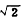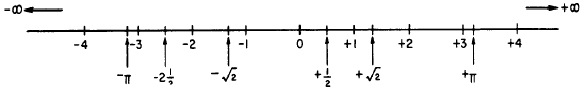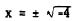Chapter 15 - Complex NumbersCustom SearchCHAPTER 15 COMPLEX NUMBERS In certain calculations in mathematics and related sciences, it is necessary to perform operations with numbers unlike any mentioned thus far in this course. These numbers, unfortunately called "imaginary" numbers by early mathematicians, are quite useful and have a very real meaning in the physical sense. The number system, which consists of ordinary numbers and imaginary numbers, is called the COMPLEX NUMBER system. Complex numbers are composed of a "real" part and an "imaginary" part. This chapter is designed to explain imaginary numbers and to show how they can be combined with the numbers we already know. REAL NUMBERS The concept of number, as has been noted in previous chapters, has developed gradually. At one time the idea of number was limited to positive whole numbers. The concept was broadened to include positive fractions; numbers that lie between the whole numbers. At first, fractions included only those numbers which could be expressed with terms that were integers. Since any fraction may be considered as a ratio, this gave rise to the term RATIONAL NUMBER, which is defined as any number which can be expressed as the ratio of two integers. (Remember that any whole number is an integer.) It soon became apparent that these numbers were not enough to complete the positive number range. The ratio, p, of the circumference of a circle to its diameter, did not fit the concept of number thus far advanced, nor did such numbers asandAlthough decimal values are often assigned to these numbers, they are only approximations. That is, li is not exactly equal to 22/7 or to 3.142. Such numbers are called IRRATIONAL to distinguish them from the other numbers of the system. With rational and irrational numbers, the positive number system includes all the numbers from zero to infinity in a positive direction. Since the number system was not complete with only positive numbers, the system was expanded to include negative numbers. The idea of negative rational and irrational numbers to minus infinity was an easy extension of the system. Rational and irrational numbers, positive and negative to ± infinity as they have been presented in this course, comprise the REAL NUMBER system. The real number system is pictured in figure 15-1. OPERATORS As shown in a previous chapter, the plus sign in an expression such as 5 + 3 can stand for either of two separate things: It indicates the positive number 3, or it indicates that +3 is to be added to 5; that is, it indicates the op- eration to be performed on +3. Likewise, in the problem 5 - 3, the minus sign may indicate the negative number -3, in which case the operation would be addition; that is, 5 + (-3). On the other hand, it may indicate the sign of operation, in which case +3 is to be subtracted from 5; that is, 5 - (+3).  Thus, plus and minus signs may indicate positive and negative numbers, or they may indicate operations to be performed.Figure 15-1. -The real number system. IMAGINARY NUMBERS The number line pictured in figure 15-1 represents all positive and negative numbers from plus infinity to minus infinity. However, there is a type of number which does not fit into the picture. Such a number occurs when we try to solve the following equation:Notice the distinction between this use of the radical sign and the manner in which it was used in chapter 7. Here, the ± symbol is included with the radical sign to emphasize the fact that two values of x exist. Although both roots exist, only the positive one is usually given. This is in accordance with usual mathematical convention. The equation raises an interesting question:What number multiplied by itself yields -4 ? The square of -2 is +4. Likewise, the square of +2 is +4. There is no number in the system of real numbers that is the square root of a negative number. The square root of a negative number came to be called an IMAGINARY NUMBER When this name was assigned the square roots of negative numbers, it was natural to refer to the other known numbers as the REAL numbers.Integrated Publishing, Inc. - A (SDVOSB) Service Disabled Veteran Owned Small Business